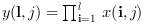Scilab Home page | Wiki | Bug tracker | Forge | Mailing list archives | ATOMS | File exchange
Scilab 6.0.1
Change language to: Français - Português - 日本語 - Русский

See the recommended documentation of this function

Scilab Help >> Elementary Functions > Matrix operations > cumprod

# cumprod

cumulative product of array elements

### Syntax

`y=cumprod(x)`
`y=cumprod(x,orientation)`
`y=cumprod(x,outtype)`
`y=cumprod(x,orientation,outtype)`

### Arguments

x

an array of reals, complex, booleans, polynomials or rational fractions.

orientation

it can be either

• a string with possible values `"*"`, `"r"`, `"c"` or `"m"`

• a number with positive integer value

outtype

a string with possible values `"native"` or `"double"`.

y

scalar or array

### Description

For an array `x`, `y=cumprod(x)` returns in the scalar `y` the cumulative product of all the elements of `x`.

`y=cumprod(x,orientation)` returns in `y` the cumulative product of `x` along the dimension given by `orientation`:

• if `orientation` is equal to 1 or "r" then:or• if `orientation` is equal to 2 or "c" then:or• if `orientation` is equal to n then:• `y=cumprod(x,"*")` is equivalent to `y=cumprod(x)`

• `y=cumprod(x,"m")` is equivalent to `y=cumprod(x,orientation)` where `orientation` is the index of the first dimension of `x` that is greater than 1. This option is used for Matlab compatibility.

The `outtype` argument rules the way the product is done:

• For arrays of floats, of polynomials, of rational fractions, the evaluation is always done using floating points computations. The `"double"` or `"native"` options are equivalent.

• For arrays of integers,

if `outtype="native"` the evaluation is done using integer computations (modulo 2^b, where b is the number of bits used),

if `outtype="double"` the evaluation is done using floating point computations.

The default value is `outtype="native"`.

• For arrays of booleans,

if `outtype="native"` the evaluation is done using boolean computations (* is replaced by &),

if `outtype="double"` the evaluation is done using floating point computations (%t values are replaced by 1 and %f values by 0).

The default value is `outtype="double"`.This function applies, with identical rules to sparse matrices

### Examples

```A=[1,2;3,4];
cumprod(A)
cumprod(A,1)

I=uint8([2 95 103;254 9 0])
cumprod(I) //native evaluation
cumprod(I,"double")
cumprod(I,2,"double")

s=poly(0,"s");
P=[s,%i+s;s^2,1];
cumprod(P),
cumprod(P,2)

B=[%t %t %f %f];
cumprod(B) //evaluation in float
cumprod(B,"native") //similar to and(B)```